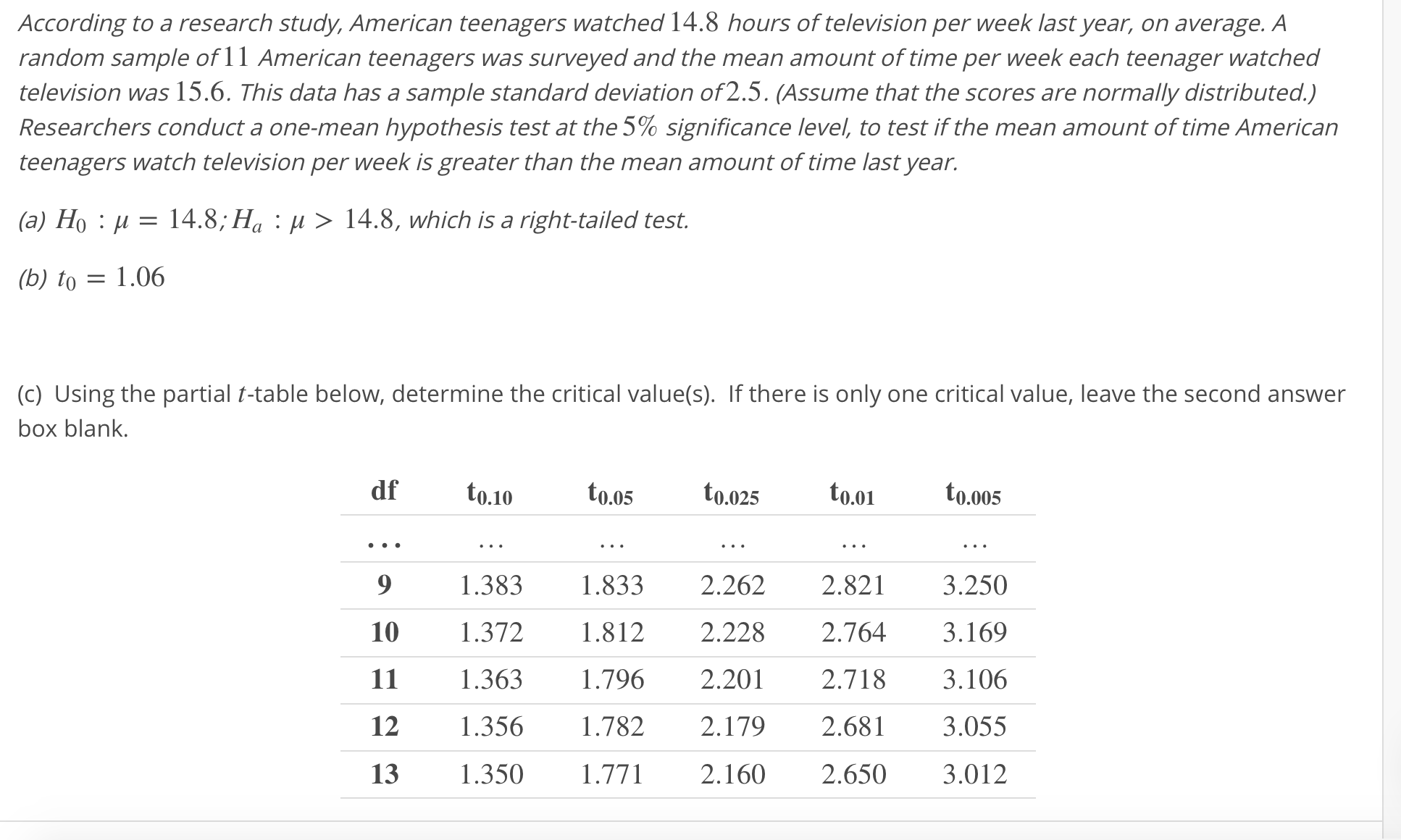# According to a research study, American teenagers watched 14.8 hours of television per week last year, on average. Arandom sample of 11 American teenagers was surveyed and the mean amount of time per week each teenager watchedtelevision was 15.6. This data has a sample standard deviation of2.5. (Assume that the scores are normally distributed.)Researchers conduct a one-mean hypothesis test at the 5% significance level, to test if the mean amount of time Americanteenagers watch television per week is greater than the mean amount of time last year.(a) Ho : μ-14.8; Ha : μ > 14.8, which is a right-tailed test.(b) to 1.06c) Using the partial ttable below, determine the critical value(s). If there is only one critical value, leave the second answerbox blank.df o.10 0.05 0.025 . 0.0059 1.383 1.8332.2622.821 3.25010 1.372 1.812 2.228 2.764 3.16911 1.363 1.7962.201 2.7183.10612 1.356 1.782 2.179 2.68 3.05513 1.350 1771 2.160 2.650 3.012

Question
1 views

Critical value(s) = ___ and ____help_outlineImage TranscriptioncloseAccording to a research study, American teenagers watched 14.8 hours of television per week last year, on average. A random sample of 11 American teenagers was surveyed and the mean amount of time per week each teenager watched television was 15.6. This data has a sample standard deviation of2.5. (Assume that the scores are normally distributed.) Researchers conduct a one-mean hypothesis test at the 5% significance level, to test if the mean amount of time American teenagers watch television per week is greater than the mean amount of time last year. (a) Ho : μ-14.8; Ha : μ > 14.8, which is a right-tailed test. (b) to 1.06 c) Using the partial ttable below, determine the critical value(s). If there is only one critical value, leave the second answer box blank. df o.10 0.05 0.025 . 0.005 9 1.383 1.8332.2622.821 3.250 10 1.372 1.812 2.228 2.764 3.169 11 1.363 1.7962.201 2.7183.106 12 1.356 1.782 2.179 2.68 3.055 13 1.350 1771 2.160 2.650 3.012 fullscreen
check_circle

Step 1

The given test is a left tailed test.

The level of significance is 5%.

That is, α = 0.05.

For one tailed test, the critical value is,

Step 2

Here, the sample size n = 11.

The degrees of freedom n-1 = 11-1 =10 and α = 0.05.

From the given table,

• Locate the degrees of freedom 10.
• Locate the value t0.05.
• Th...

### Want to see the full answer?

See Solution

#### Want to see this answer and more?

Solutions are written by subject experts who are available 24/7. Questions are typically answered within 1 hour.*

See Solution
*Response times may vary by subject and question.
Tagged in

### Hypothesis Testing